# A Decentralized Lie Detector

## by Jack Peterson

Barack Obama won the 2012 U.S. Presidential election.
We know this is true, because the election's already been run, and the winner is known. You know it; I know it. But, the blockchain doesn't know it. Blockchains don't know anything about the external world, except what we tell them. So, how do we tell the blockchain who won the Presidential election?
Since everyone knows the answer, one really easy way to get the election results into the blockchain is to just ask people about it: "Who won the election?" People punch in the winner's name, and now the blockchain is knowledgeable and happy.
But, what if people don't all tell the blockchain the same thing?
Well, if they're honest, they should! After a Presidential election, it's really, really easy to find out who the winner was: you probably already know it, and if you don't, you just type "US President election winner" into Google, and, half a second later, you've got the answer. Furthermore, if I ask you and 100 other people, I shouldn't get 100 or even 10 different answers; if you're all honest, you should all say the same thing.
The trickier question is, what if people aren't honest? Suppose you're in a classroom with 50 honest people and 50 lazy jerks. If you ask the group, "What is the temperature outside right now?", the 50 honest people will look at the thermometer you've conveniently hung outside -- which reads 65 degrees Fahrenheit -- and all report that the temperature is 65 degrees Fahrenheit. The 50 lazy jerks are too lazy to check the thermometer, so they just make up a number. Notice that it's easy to figure out what the correct answer is in this case: the lazy jerks very likely did not all pick the same number, so the most common answer is most likely to be correct. This is our guiding light for lie detection:
Lies are more variable than truths.
Taking this statement at face value, we could design a system that sifts out truths from lies by just accepting the most common answer as correct. In fact, this is the basic idea behind Schellingcoin, which takes the median answer to be correct. The difficulty with the Schellingcoin mechanism is that (as its author, Vitalik, notes) it is susceptible to collusion attacks. The simplest attack is if a group of dishonest users controls 51% of the coins, they control 100% of the outcomes. For example, suppose 51/100 users had a million dollars riding on Mitt Romney, which would be one hell of an incentive for them to make the consensus algorithm declare Romney to be the winner.
What if the dishonest group controls 51% of the reports, but they vote on many events at the same time? Before, each user's answer was just a number. Now each user's answers is not just a number, but a series of numbers -- a signal -- which has a value for every event. There might be a 51% group of disgruntled Romney supporters willing to lie about the election, but what if there were 19 other events they had to simultaneously report on? The chances are much lower that the members of this dishonest group are willing and able to perfectly coordinate their lies across so many different events!
In other words, "lies are more variable than truths" is even more true if we consider the users' reports across many events. Put another way, by increasing the number of times each voter's signal is sampled, we greatly increase the resolving power of the Schellingcoin idea. This idea is the foundation of Augur's event resolution mechanism (as well as that of Truthcoin, Augur's theoretical inspiration).

In Augur, truth equals consensus. As such, the Augur oracle is intended to be used for events which are easily and objectively determinable after the event has occurred. (For example, the winner of an election.)  However, there are many cases where consensus might not reflect the truth, such as events where

• ...there is ongoing controversy about what happened (e.g., "Malaysia Airlines Flight 370 was brought down by terrorists")
• ...the outcome is subjective (e.g., "was Carter a good President?")
• ...the outcome is unreasonably difficult to determine (e.g., "what is President Obama's checking account balance?")

These questions are not good candidates for Augur!  In fact, all questions include a "this was a bad question" answer, in case a user is asked to report on an ill-defined or unanswerable event.

(Of course, Augur's oracle can dutifully report the consensus in these cases -- and the consensus very often will be "this was a bad question" -- but it is up to you the user to use your judgment as to whether this consensus is an accurate reflection of the truth.)

Note: The following sections are a bit technical. If you're not familiar with the concepts of eigenvalues and eigenvectors, here are a few good primers on eigenvalues, eigenvectors, and how they're used in Principal Component Analysis:
Searching for lies in the matrix
Here is an example table of reports, in which each user's answers are a row, and each column represents an event:There are three questions consensus needs to address:
1. How do you determine the amount that each user contributed to the overall variability in this table?
2. How do you determine what the correct event outcome actually was?
3. How do you incentivize people to report honestly?
First, let's nail down exactly what we mean by "variability". In statistics, variance quantifies how widely dispersed a set of values is; i.e., if most users agree on an event's outcome, that event has a low variance. Covariance is how much two sets of values (i.e., two events) vary together. So, roughly speaking, "variability" is variance + covariance -- this isn't perfect, but let's roll with it for now.
Reputation -- a tradeable token -- is needed to report on events.  Each user's report is weighted by the number of Reputation tokens the user has.  Reports are made on events (after they expire) at fixed, pre-defined intervals.  (In this simple example, each user starts with a single Reputation token.)
Suppose there are E total events being reported on by N users, and let r be a column vector of Reputations. We first take the table B of reports and construct its weighted covariance matrix, where the weights are Reputations. The centered table (the table with each event's weighted mean value subtracted out) X is:where 1 is a column vector of ones and T denotes the transpose operation (swapping columns and rows). The ordinary (unweighted) covariance matrix is given by multiplying the centered data matrix and its transpose; similarly, the weighted covariance matrix is:where R has the elements of r on its diagonal and zeros elsewhere,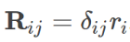Each row (and column) in Σ corresponds to the variability across users, within a single event. Since there are E events, Σ is an E x E matrix. Next, we diagonalize Σ,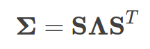revealing Λ, an E x E matrix with Σ's eigenvalues on its diagonal, and zeros elsewhere: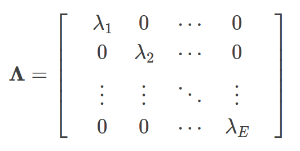The eigenvalues are in descending order, λ_i > λ_(i+1).
The eigenvector s_1 associated with the largest eigenvalue λ_1 is the principal component, which is a unit vector oriented in the direction of as much of the report matrix's variability as can be captured in a single dimension. This direction is a new coordinate axis. By projecting the (centered) data onto this axis, we obtain a non-conformity score for each user: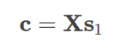Score c_i of this vector then tells us user i's contribution to the direction of maximum variance. Using these scores for event consensus is called Sztorc consensus (named after its ruthlessly strategic and paranoid inventor, Paul Sztorc). These scores are then used to reward users who reported with the consensus (low scores) and punish those who voted against it (high scores).Shown here is the user's non-conformity score (on the x-axis) versus their gain (or loss) in Reputation (on the y-axis). Blue indicates users that told the truth; red indicates dishonest users. The size of the circle indicates the number of users that reported identical results. As expected, by far the largest single circle is that of the honest users, and (in this simple example) those users were rewarded accordingly, while the dishonest users all lost Reputation.
The second eigenvector, and beyond!
For large and/or complicated ballots, intuitively, you might expect to need more than a single direction to really capture what's going on in the ballot. If there are in fact multiple directions required -- multiple coordinate axes -- then we need to extract more than just the first eigenvector from the covariance matrix.
Empirically (in simulations, anyway), this seems to be the case. Often the first eigenvector accounts for 40% or less of the total ballot variance, as shown in this breakdown for the simple example shown above: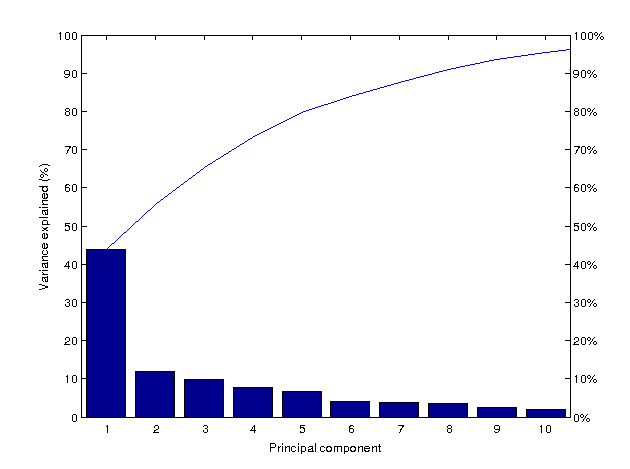In this plot, the bars represent the percentage of variance explained by each component. The blue line is the cumulative percentage explained. This plot also suggests a simple way of incorporating more directions into our results: just keep adding eigenvectors until we reach some pre-defined threshold!
Setting a fixed variance threshold (α) allows us to extract the number of components (n) needed to explain at least α * 100% of the report matrix's total variance. The non-conformity vector c is calculated by summing together the projections onto the first n components, each of which is weighted by its eigenvalue λ:This is the fixed-variance threshold method, first described in the Augur whitepaper. Although this method has improved resolving power (see below), it has the downside of being more computationally demanding than Sztorc consensus, as it requires the computation of the first n eigenvectors.
However, we're really not interested in the eigenvector basis per se: in fact, we only care about the scores. The scores tell you how much each user contributed to the direction of maximum variance.
The eigenvectors -- the new coordinate axes -- are obtained by diagonalizing the per-event covariance matrix, which is an E x E matrix, where rows/columns account for each event's covariance across all users. But, if all we care about is each user's relative contribution to the overall variability, this information is available more directly by constructing the per-user covariance matrix:where the row-centered report matrix is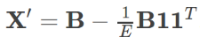Σ' is an N x N matrix, where each row/column represents a user's covariance, taken across all events. Summing across this matrix's rows, each user's contribution to the total variance and covariance is found as the ratio of that user's sum to the total:Test driving the algorithms
A valuable rule-of-thumb I picked up in graduate school: when in doubt, simulate!
The way I'm testing and comparing different event resolution algorithms is using Monte Carlo simulations. If you're not familiar with Monte Carlo, it basically means: create a bunch of randomized datasets (with known outcomes), feed them through the consensus machinery, and see what comes out the other side.
First, create a vector of known answers (to True, False, Indeterminate questions) with random entries. There are two kinds of reporters:
1. Users can be honest, in which case they just copy the correct answers down.
2. Or dishonest/lazy/ignorant, in which case they just pick answers at random on their ballot.
Dishonest users also have a small -- in this example, 30% -- chance to copy one another. (This is an adjustable parameter, γ = 0.3.) Imagine that the dishonest/lazy/ignorant user calls up their buddy and says, "Hey Bob, can you just send a screenshot of your ballot over, I don't feel like filling this stupid thing out, I could be training for the upcoming hot dog eating contest instead." Dishonest users also have a γ^2 = 0.09 (9%) chance to copy in triples, and a γ^3 = 0.027 (2.7%) chance to copy in quadruples.
First, here are the results of a set of 500 simulations, for the three event resolution algorithms discussed so far in this post:
1. Sztorc, which uses only the users' scores on the largest eigenvector.
2. Fixed-variance is a weighted sum of eigenvectors up to a fixed threshold of 90% variance.
3. Covariance uses ratios from the per-user covariance matrix, and does not require matrix diagonalization.
The first metric I used to compare the algorithms is the percentage of "beats". A "beat" is a dishonest user that received a larger reward than the honest users. In this plot, the y-axis is % of dishonest players that were beats. The x-axis is the % of dishonest players in the simulation (this is the same for all plots). For this metric, lower scores are better -- ideally, you'd want no beats at all!
Next is the "liars' bonus", i.e. the amount of bonus Reputation dishonest users received, minus the amount received by honest players. As before, lower = better:
Finally -- and in some ways the most important -- is the percentage of outcomes that the algorithm got right. (Since these are just tests, I define the "correct" answer beforehand, so I know whether the consensus lands on the correct result or not!) Here, higher percentages are better:
As expected, the fixed-variance method (yellow) significantly outperforms the Sztorc method (blue) across-the-board, due to the extra information contained in the additional eigenvectors. More surprising is that it also outperforms the covariance ratio method. This is possibly due to the eigenvalue weightings, which are not available without diagonalizing the covariance matrix.
Cokurtosis: collusion's fat tail
One of the shortcomings of a covariance-based technique is that it is based solely on second-order statistics (i.e., variance/covariance), and ignores higher-order statistics, such as skewness, kurtosis, etc. I think this could be a problem, since "fat tails" are everywhere in social systems, because herd mentality = positive feedback = fat tails.
In particular, the kind of cheating we're most concerned about is collusion, which is essentially just users copying each other's ballots. My intuition is that this copying is very likely to manifest as a sort of "rich get richer" behavior: the more users have copied a particular ballot's answers, the easier it is for still more users to find -- and therefore copy -- the ballot. In other words, exactly the kind of directly proportional feedback that gives rise to power-law behavior in loads of different systems.
If this is right, then this sort of cheating/collusion should create excess among-user kurtosis/cokurtosis -- i.e., they should be contributing extra to the fourth joint central moment ("cokurtosis") tensor. This means that you can sum across the tensor's fibers and score the users in the same way that you can directly from the covariance matrix.
To investigate this, I put together a small Julia library for building joint central moment tensors, JointMoments.jl, and conducted the same tests as above (for the second-order-only methods):
So far, it seems like this cokurtosis tensor-based method has better resolving power than any of the other methods! But, this is just the result of a small preliminary simulation -- lots more testing to do before this method is declared the winner for real.
One thing that will be important is throwing more (and different) "liar" patterns at each algorithm and seeing how they hold up. Right now, the "dishonesty" in the simulations is fairly tame, and really is intended to capture apathy/laziness more than actual focused attack attempts.
My prediction? Given the winners of the above comparisons, I suspect the best results will be from combining the cokurtosis and fixed-variance threshold methods.
All the simulations and algorithms discussed here are 100% open-source, so if you have suggestions, criticism, or just want to jump in and start tinkering, please do!
• pyconsensus: standalone Python implementation of Augur's oracle
• Simulator.jl: numerical simulations, statistics and plotting tools
Originally posted at The Ramble.
Edited on 3/4/2015 to include a short discussion of what Reputation is, as well as the kinds of events that Augur's oracle is meant to address.
All Posts
×

Almost done…

We just sent you an email. Please click the link in the email to confirm your subscription!